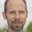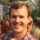Calculation Custom Field Type

The use case is that you create a custom table for storing financial/budgeting type data for a project. You add a number custom field for "Planned Spend" and another number custom field for "Actual Spend". The desire is to have another calculated field named "Spend Delta" that would be derived based on a calculation of "Planned Spend" - "Actual Spend" which would be specified as part of the "Calculation" type properties. Similarly, you might want to have a derived custom field that displays the % of Budget Spent so the custom field properties would allow you to specify the equation is "Actual Spend"/"Planned Spend".

I used a custom table example but it should be available as a general custom field option. But, it seems especially compelling to be able to perform operations between different fields in a custom table.

•Matt Case
• Dec 16 2016
• Shipped
 Release time frame
• Feb 6, 2019

It is now possible to create calculated fields with worksheets.

Think of worksheets in Aha! as two-column spreadsheets. You can create as many rows as you need and they automatically calculate data. You can use worksheets to do basic math — add, subtract, multiply, and divide. But you can also perform more complex functions, such as averaging or calculating the time between two dates.

With this initial release, worksheet fields can reference any other worksheet field. We are actively working on an enhancement that would allow worksheets to reference any other field in Aha! We will keep this idea updated as we make progress there.

•Wayne Allan commented
December 30, 2016 10:57

It would be good also if it had some basic functions such as COUNT(), AVERAGE(), MAX() and MIN()

•Guest commented
March 15, 2017 23:53

I would suggest a % complete based on any numeric entries (beyond budget) for example, the ability to gain the % complete of done requirements mapped under a feature, or ToDos mapped under a requirement.

•Marc Seaberg commented
April 13, 2017 16:43

My use case is to have a calculated field for the feature score. For example, we're planning to use multiple "scorecards" for each feature  - a set of questions around consumer need, a different one for financials, another for strategic fit, etc. Each of these should roll up to a single calculated score.

•Guest commented
May 04, 2017 13:42

I'd like to suggest to have the planned - and actual spend per release/sprint and that those can be summed up to the "total planned spend" or "total actual spend".

•Bruce Cannon commented
August 08, 2017 17:45

In addition to the other excellent comments here, I'd add that a formula field which can reference any other custom field or system field should be available everywhere.

As an example, I'd like to have a bubble chart whose bubble's color are determined by a score.  But the bubble color can only take a text value as input.  So, I'd like to work around that limitation by using a formula field for my scorecard's final score, in which the numeric total is used as an offset into a list of text values.  Or simpler, leave the scorecard alone but use it's numeric score in a formula field which does this lookup, and then assign that field as the input to the bubble chart.

•Devik Lansing commented
August 09, 2017 18:28

AGree that we would need operations on custom fields and tables.  We really really need calculated fields in general.

•Daniel Scudds commented
August 15, 2017 00:04

Definitely second this requirement. This functionality is required to generate charts based on the data from lists.

•Guest commented
October 24, 2017 07:12

Roll up of Product Level information to the Product Line/Portfolio/Company Level. Suppose I am entering the size for a particular Product / Feature then it should roll up to the Product Line or Portfolio or Company level.

•Jennifer Booker commented
November 08, 2017 23:09

It would be nice to have the ability to have logic functions IF and SUMIFS

•Alex HOFFMAN commented
November 30, 2017 14:18

Not having the ability to calculate (particularly subtract, add, and divide) is killing me. I have a lot of fields that need to be divided by other fields or a static number. Without the ability to do calculations in Aha! I'm forced to add these calculations into my source data when importing from our legacy system. Not ideal.

•Alex HOFFMAN commented
December 12, 2017 20:07

I'm buying my team calculators for Christmas so they can do addition, subtraction, and division of a few of their fields in Aha each week.

•John Seifert commented
January 10, 2018 17:31

I totally need this ability.  Support suggest using Pivot tables.  That provides basic functionality, the calculations meant here are needed.  Also, I do not want custom table to make this functionality to work.  This should used on a list view/report.

•April 16, 2018 15:41

It would be helpful to also be able to access metrics from within scorecards as part of the custom field formula builder. One would then be able to calculate certain values such as "Complexity" which may be made up of a set of metrics within a scorecard vs. Effort which may be made up of another set of metrics within the same scorecard.

•Julie Edwards commented
June 29, 2018 14:33

This would be SO handy!  Please implement this soon!

•Dan Eisenhut commented
August 22, 2018 19:32

Definitely needed!  Please make sure that it handles using a custom text string as a variable when the string includes symbols, such as a percent sign (e.g. "57%").

•Leanne Miller commented
September 11, 2018 14:13

I need this as well and need to be able to create IF statements.   I need to report on whether Ideas are created Internally or Externally.  I would like to create a calculated field that would look at the Submitted by Domain and determine Internal if the domain is our company domain or blank (Ideas created inside of Aha leave that blank) and External if it’s anything else.

•Matt Grastorf commented
September 11, 2018 17:09

Ability to for conditional formulas in custom fields would be great also,  Like If "Release Date" <= Today, Custom Field = "OverDue"

•Justin Reed commented
October 04, 2018 22:05

Happy to pile on here. Ultimately, I have to export to Excel to get some of the functionality I need. I already fight with my teams to get out of Excel and into Aha, so it's unfortunate I have to tell them to use Excel to get calculations (most frequently used: date diff, basic arithmetic, average, min, max, count, if/else). We need calculations for a number of reasons, but like other commenters, we use them most when estimating and budgeting.

•Henrik Lund Binderup commented
November 16, 2018 13:26

I have a task to work with 3 point estimations using Aha! To secure I have my data in AHA as long as possible instead of exporting to excel.

I have added this calculation E = (a + 4m + b) / 6 in a scorecard for each team making the 3 point estimate.

When this estimates are typed into the scorecards I have made a pivot table to be able to sum the estimates for each feature and for each team.

But how do I get the calculation of the standard diviation visualized using this calculation. SD = (b − a) / 6

I would like to have a tool similar to what is available in the scorecard to make my individual calculations.

From Wikipidia

https://en.wikipedia.org/wiki/Three-point_estimation

The three-point estimation technique is used in management and information systems applications for the construction of an approximate probability distribution representing the outcome of future events, based on very limited information. While the distribution used for the approximation might be a normal distribution, this is not always so and, for example a triangular distribution might be used, depending on the application.

In three-point estimation, three figures are produced initially for every distribution that is required, based on prior experience or best-guesses:

• a = the best-case estimate
• m = the most likely estimate
• b = the worst-case estimate

These are then combined to yield either a full probability distribution, for later combination with distributions obtained similarly for other variables, or summary descriptors of the distribution, such as the meanstandard deviation or percentage points of the distribution. The accuracy attributed to the results derived can be no better than the accuracy inherent in the 3 initial points, and there are clear dangers in using an assumed form for an underlying distribution that itself has little basis.

Estimation

Based on the assumption that a double-triangular distribution[clarification needed] governs the data, several estimates are possible. These values are used to calculate an E value for the estimate and a standard deviation (SD) as L-estimators, where:

E = (a + 4m + b) / 6

SD = (b − a) / 6

E is a weighted average which takes into account both the most optimistic and most pessimistic estimates provided. SD measures the variability or uncertainty in the estimate. In Project Evaluation and Review Techniques (PERT) the three values are used to fit a PERT distribution for Monte Carlo simulations.

The triangular distribution is also commonly used. It differs from the double-triangular by its simple triangular shape and by the property that the mode does not have to coincide with the median. The mean (expected value) is then:

E = (a + m + b) / 3.

In some applications, the triangular distribution is used directly as an estimated probability distribution, rather than for the derivation of estimated statistics.

•William Orme commented
December 20, 2018 16:04

My use case is for calculated scorecard off custom fields and Feature fields such as Estimate, so we can run algorithms based on data collected in Salesforce, Zendesk and Jira to give auto-updating scores.

•David Desrosiers commented
06 Feb 17:06

Sorry I'm a bit confused, is this released or in progress?
I see contradictory messages
Status is "Shipped" and announcement "It is now possible"

but it is also labeled with "Release time frame 1 month"

Thanks

•